This tutorial will demonstrate how to perform a VLOOKUP from another worksheet or spreadsheet in Excel and Google Sheets. If your version of Excel supports XLOOKUP, we recommend using XLOOKUP instead.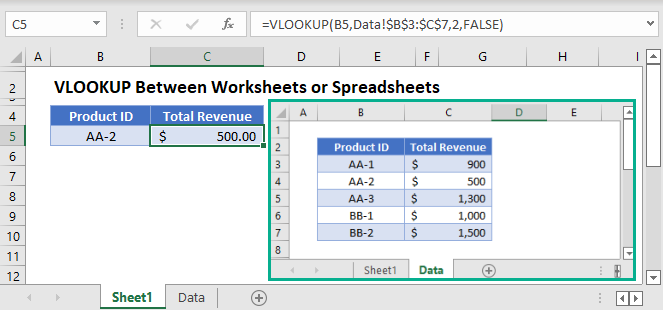## VLOOKUP from Another Worksheet

To reference a range from a different sheet, click the tab name at the bottom of Excel and select your range. Excel will automatically add the appropriate cell reference:

``=VLOOKUP(B3,Data!\$B\$3:\$C\$7,2,FALSE)``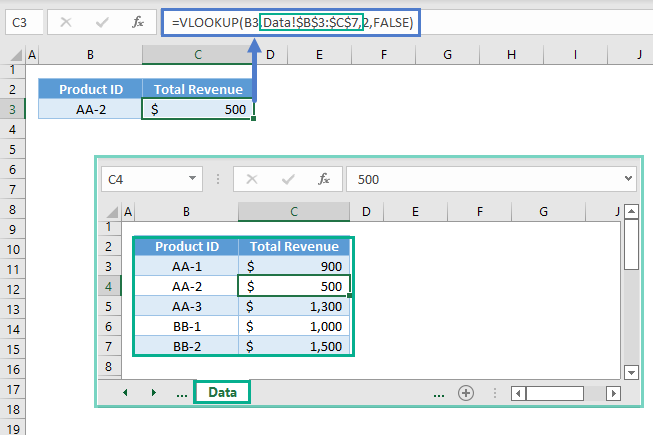If you’d like, you can manually type the cell reference. Notice how it’s displayed above.

However, this reference format will not work if there are spaces or special characters. If there are spaces or special characters, the cell reference will look like this instead (notice the apostrophes):

``=VLOOKUP(B3,'Data - 2'!B3:C7,2,FALSE)``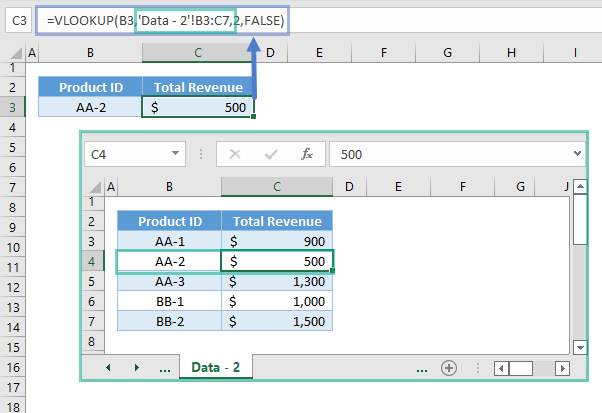## VLOOKUP from Another Sheet – Google Sheets

The same rules also apply to Google Sheets: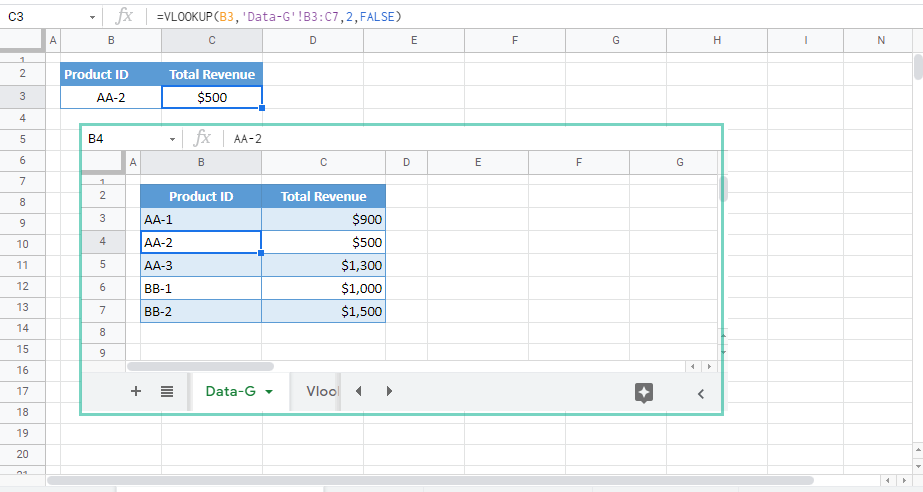## VLOOKUP from Another Workbook

If the data set is stored in a sheet from another workbook, we must also add the workbook reference.

``=VLOOKUP(B3,[WorkbookV.xlsx]Data!\$B\$3:\$C\$7,2,FALSE)``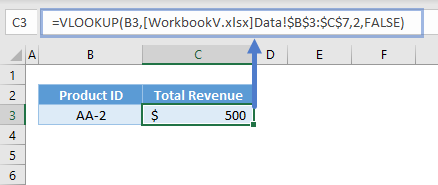If the workbook is open, only the workbook name will be displayed. When the workbook is closed, the full path will be shown:

``=VLOOKUP(B3,'D:\AUTOMATE EXCEL\[WorkbookV.xlsx]Data'!\$B\$3:\$C\$7,2,FALSE)``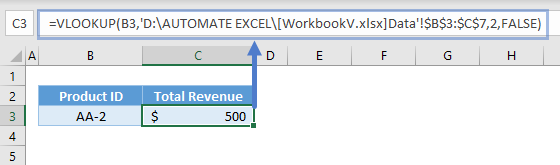## VLOOKUP from Another Google Sheets

In Google Sheets, we can nest the IMPORTRANGE Function in VLOOKUP to import the data set from other Google spreadsheets.

``=VLOOKUP(B3,IMPORTRANGE("https://docs.google.com/spreadsheets/d/15g8Wu0GDfKsYKmqUPsrMSxziz0VHQliBol0tYC1vVjs/edit?usp=sharing","Data-G!B3:C7"),2,FALSE)``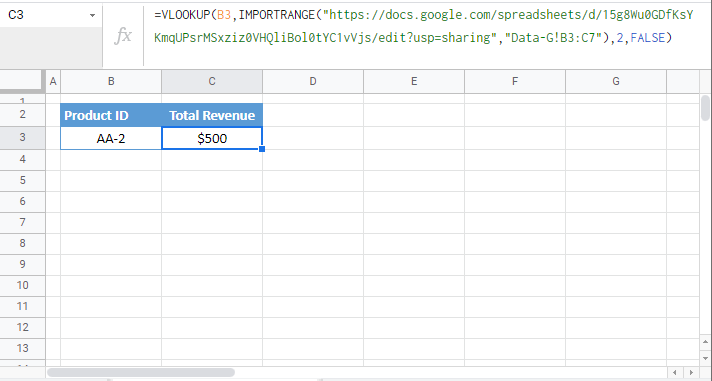Note: IMPORTRANGE Function has two arguments, which are both inputted as texts. The 1st argument is the URL of the spreadsheet, and the 2nd argument is the reference. The reference can be both the sheet and range or range only. If range only, it will use the first sheet as the sheet reference by default.

The IMPORTRANGE requires permission to pull data. Once access is granted, it won’t ask for permission again.

### Excel Practice Worksheet

Practice Excel functions and formulas with our 100% free practice worksheets!